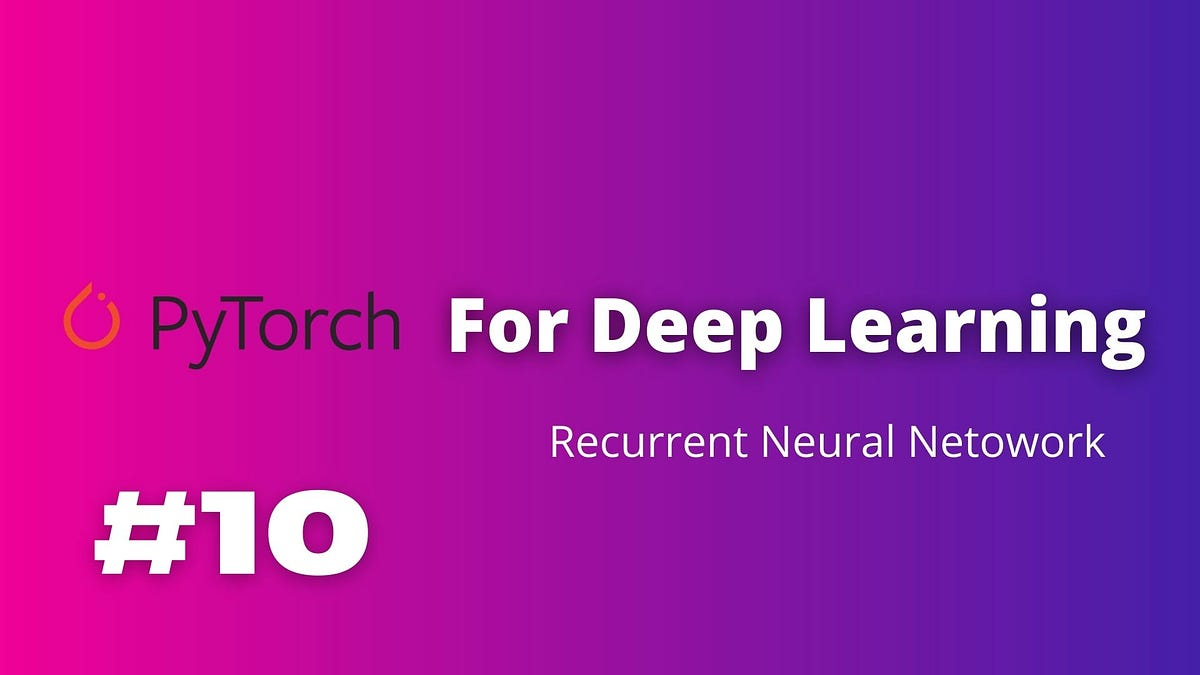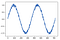# PyTorch for Deep Learning — LSTM for Sequence DataPyTorch for Deep Learning — LSTM for Sequence Data. Time-series data changes with time. In this article, we'll be using PyTorch to analyze time-series data and predict future values using deep learning.

## Note

sorry for misspelling network , lol.

All the code files will be available at : https://github.com/ashwinhprasad/PyTorch-For-DeepLearning

## What are RNNs and LSTMs ?

Recurrence Neural Network are great for Sequence data and Time Series Data. Long short-term memory is an artificial recurrent neural network architecture used in the field of deep learning. LSTMs and RNNs are used for sequence data and can perform better for timeseries problems.

An LSTM is an advanced version of RNN and LSTM can remember things learnt earlier in the sequence using gates added to a regular RNN. Both LSTM’s and RNN’s working are similar in PyTorch. So, once we coded the Lstm Part, RNNs will also be easier to understand.

In this notebook, we are going to try and predict a sinewave with a Recurrence Neural Network.

Theory for RNNs and LSTMs will not be covered by this post. This is only for pytorch implementation of rnn and lstm.

## Jumping to the Code :

1. Importing the Libraries
``````#importing the libraries
import numpy as np
import torch
import matplotlib.pyplot as plt``````

2. Data Pre-processing

I am creating a sinewave and as I already said, lstm takes sequence inputs.So, The input would be like the following :

Input : [point 1, point 2, point 3…..,point n] prediction : [point n+1].

we need many rows like this to create the dataset.

``````#creating the dataset
x = np.arange(1,721,1)
y = np.sin(x*np.pi/180)  + np.random.randn(720)*0.05
plt.plot(y)
``````## PyTorch for Deep Learning | Data Science | Machine Learning | Python

PyTorch for Deep Learning | Data Science | Machine Learning | Python. PyTorch is a library in Python which provides tools to build deep learning models. What python does for programming PyTorch does for deep learning. Python is a very flexible language for programming and just like python, the PyTorch library provides flexible tools for deep learning.

## PyTorch for Deep Learning | Data Science | Machine Learning | Python

PyTorch is a library in Python which provides tools to build deep learning models. What python does for programming PyTorch does for deep learning.

## 50 Data Science Jobs That Opened Just Last Week

Data Science and Analytics market evolves to adapt to the constantly changing economic and business environments. Our latest survey report suggests that as the overall Data Science and Analytics market evolves to adapt to the constantly changing economic and business environments, data scientists and AI practitioners should be aware of the skills and tools that the broader community is working on. A good grip in these skills will further help data science enthusiasts to get the best jobs that various industries in their data science functions are offering.

## Applications Of Data Science On 3D Imagery Data

The agenda of the talk included an introduction to 3D data, its applications and case studies, 3D data alignment and more.

## PyTorch for Deep Learning

PyTorch is a library in Python which provides tools to build deep learning models. What python does for programming PyTorch does for deep learning.